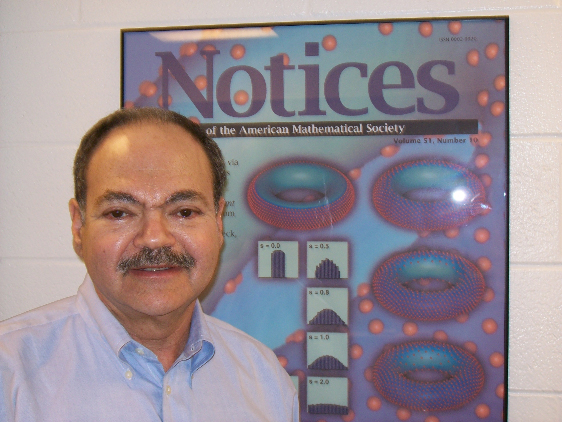# Consortium Distinguished Lecture Series

## February 9, 6:00 pm (EET, Sofia time) ICMS-Sofia, via Zoom## Sampling with Minimal Energy: A talk in commemoration of Bl. Sendov’s 90th birth date.

Minimal discrete energy problems arise in a variety of scientific contexts – such as crystallography, nanotechnology, information theory, and viral morphology, to
name but a few.  Our goal is to analyze the structure of configurations generated by optimal (and near optimal) $N$-point configurations that minimize the Riesz s-energy over a bounded surface in Euclidean space. The Riesz s-energy potential, which is a generalization of the Coulomb potential, is simply given by $1/r^s$, where $r$ denotes the distance between pairs of points. We show how such potentials and their minimizing point configurations are ideal for use in sampling surfaces. Connections to the breakthrough results by Viazovska, Cohn, et al on best-packing and universal optimality in 8 and 24 dimensions will be discussed. Finally we analyze the minimization of a $k$-nearest neighbor truncated version of Riesz energy that reduces the order $N^2$ computation for energy minimization to order $N log N$, while preserving global and local properties.